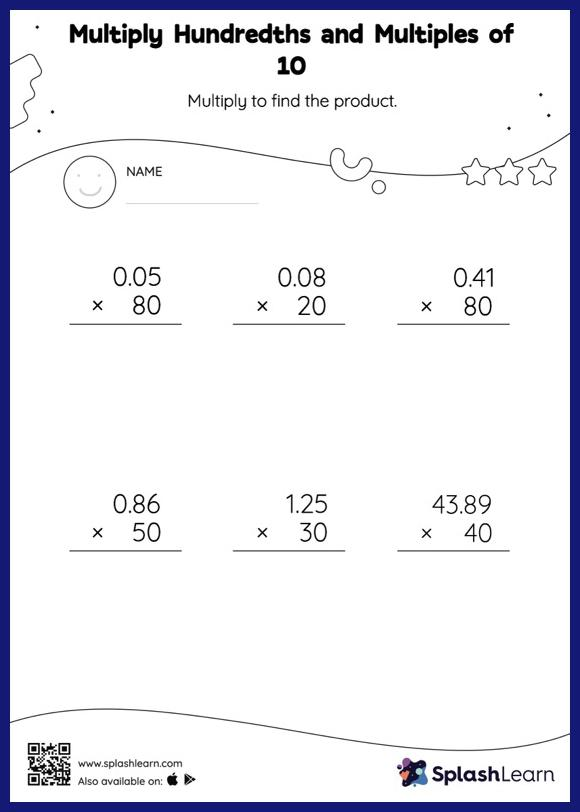# Multiply Hundredths and Multiples of 10: Vertical Multiplication Worksheet

Home > Multiply Hundredths and Multiples of 10: Vertical MultiplicationMultiply hundredths and multiples of 10 worksheet is a great way to help learners become fluent with the concept of multiplication. Students understand that multiplying a decimal by a number that ends in zeros causes the decimal point to be placed in the product as many places from the right as the number of decimal digits in the multiplicand. In multiply hundredths and multiples of 10 worksheet, they practice this idea in great detail. In each problem, the numbers are laid out in the vertical format. In this format, pair of digits in each successive place are tackled one by one. This helps students follow a structured approach.